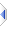# join_by

 Definition: sequence res = join_by(sequence s, integer step, integer n, object step_pad="   ", object n_pad="\n") Description: The join_by routine allows columnisation of results across the screen for easier reading. Best illustrated by example, join_by iteratively interleaves up to n blocks of size step with step_pad, before joining with n_pad, repeated as necessary up to length(s) - however, typically you would experiment with different values until finding a layout that you like, so fully "grokking" what this really does is not particularly helpful, anyway. Segments are expected to be padded to a consistent length, before this routine is called, to achieve proper alignment. Note that n_pad is duplicated when step>1 between each (n*step)-block, but only occurs once at the end, unless a trailing "" is supplied. When step=1, n_pad occurs once between each n-block, and only at the end in cases where a trailing "" has been provided. Uses join internally. The default for step_pad is three spaces. Returns a string or sequence. Example 1: ```sequence s = {"A","B","C","D","E","F","G","H","I","J","K","L"} puts(1,join_by(s,3,2)) puts(1,"=\n") -- A D -- B E -- C F -- -- G J -- H K -- I L -- = puts(1,join_by(s,2,3)) puts(1,"=\n") -- A C E -- B D F -- -- G I K -- H J L -- = puts(1,join_by(s,3,4)) puts(1,"=\n") -- (join_by(s,3,5) same) -- A D G J -- B E H K -- C F I L -- = puts(1,join_by(s,4,3)) puts(1,"=\n") -- (join_by(s,4,4) same) -- A E I -- B F J -- C G K -- D H L -- = puts(1,join_by(s,2,5)) puts(1,"=\n") -- (example 5, see the second note) -- A C E G I -- B D F H J -- -- K -- L -- = puts(1,join_by(s,5,2)) puts(1,"=\n") -- (example 6, same as join_by(s,5,3), see last note) -- A F -- B G -- C H -- D I -- E J -- -- K -- L -- = puts(1,join_by(s,1,4)) puts(1,"=\n") -- A B C D -- E F G H -- I J K L -- = puts(1,join_by(s,1,6)) puts(1,"=\n") -- A B C D E F -- G H I J K L -- = puts(1,join_by(s,1,5)) puts(1,"=\n") -- A B C D E -- F G H I J -- K L -- = ``` Obviously the puts(1,"=\n") make the output easier to follow: without them, each example starts on a new line, however blank lines only occur mid-example, but not between the examples. In each example above, both the 'A' and the '=' are actually/always the first thing on a new blank line, the "-- " have been added by me. Note how in the "heightwise" (step>1) examples 5 and 6 the trailing (partial) n-blocks retain the step-pattern, as of course does the last "widthwise" (step=1) example. Should you wish to "flatten" the trailing partial block in an otherwise heightwise invocation, I suggest you spilt s into n-wise whole sets and the rest, and invoke join_by twice, with some different step & n on the partial block(s), which could have all manner of desired shapes for the partial>step cases, and therefore it is not really feasible to automate that reliably. In the 6th example, but with n=3, you might want/expect the final K L up in column 3, however the routine as-is cannot do that. Instead, using join_by(s&repeat("",3),5,3) should achieve the desired effect, ie first padding s to a whole multiple of step. There are always going to be some edge cases where length(s) is not a whole multiple of step*n that every man and his dog expects to behave differently, and for that you really need to be prepared to roll your own bespoke version (obvs, copy and rename). Example 2: ```string s = "ABCDEFGHIJKL" puts(1,join_by(s,1,4,"")) puts(1,"=\n") -- ABCD -- EFGH -- IJKL -- = puts(1,join_by(s,1,6,"")) puts(1,"=\n") -- ABCDEF -- GHIJKL -- = ``` As above, I manually inserted the "-- ", and the "=\n" both separate examples and prove there is a '\n' after the 'L'. I should note this splits and re-joins every single individual character, so maybe if you are using join_by() on million-character+ strings it might be noticeably faster to use manual slices in a loop (but test/time to be sure any saving would be worthwhile first). Example 3: demo/rosetta/IQpuzzle.exw Implementation: See builtins\pflatten.e (an autoinclude) for details of the actual implementation. It contains some (lightly tested and) commented-out changes required to automatically output a trailing partial<=step widthwise. There may also be some partial<=(or>)n cases (where that differs from partial<=(or>)step) which merit a subtly different approach, and that is the reason why I said lightly tested and left those changes commented-out. See Also: join, columnize, shorten